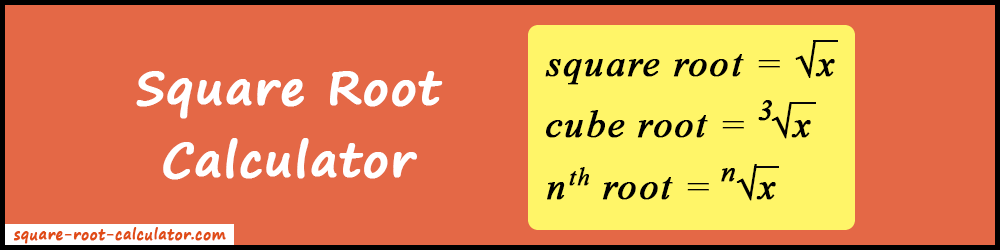# Square Root Calculator

The square root calculator is designed to calculate the square root, cube root, and nth root or radical of a given number.

3

Stuck in finding the square roots for your math homework? Make it simple and fast with our square root calculator. It can also add, subtract, multiply, and divide two square root values. In addition, you can find the square root, cube root, and nth root or radical of any given number. So, it's a one-stop solution for all types of root calculations.### What is Square Root?

A square root of a number can be defined as a number which when multiplied with itself gives that number. For example, the square root of 9 is 3. When 3 is multiplied by 3 gives 9. The radical symbol (√) is used for denoting square root. It's also known as the "Root" or "Surds".

### How to Use the Square Root Calculator?

1. Firstly, select the specific tab for which you need to find the root. There are three tabs: Square Root, Cube Root, and nth Root.
2. To perform arithmetic operations between two square roots, press the "Expand" button.
3. Now enter the required values in the input boxes and press the "Calculate" button.
4. As a result, the root of the entered number will be displayed on your screen.
5. Lastly, the "Reset" button will clear all the input and output fields and you can start the new calculation.